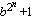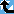# Generalized Fermat Divisors (bases 3,5,6,10,12)

The Prime Pages keeps a list of the 5000 largest known primes, plus a few each of certain selected archivable forms and classes. These forms are defined in this collection's home page.

The numbers Fb,n =(with b an integer greater than one) are called the generalized Fermat numbers. (In the Prime database they are denoted GF(b,n) to avoid the use of subscripts.) It is reasonable to conjecture that for each base b, there are only finitely many such primes.

As in the case of the Fermat numbers, many have interested in the form and distribution of the divisors of these numbers. When b is even, each of their divisors must have the form

k.2m+1
with k odd and m>n. For this reason, when we find a large prime of the form k.2n+1 (with k small), we usually check to see if it divides a Fermat number. For example, Gallot's Win95 program Proth.exe has this test built in for a few choices of b.

The number k.2n+1 (k odd) will divide some generalized Fermat number for roughly 1/k of the bases b.

###Record Primes of this Type

rankprime digitswhowhencomment
17 · 220267500 + 1 6101127 L4965 Jul 2022 Divides GF(20267499, 12) [GG]
23 · 216408818 + 1 4939547 L5171 Oct 2020 Divides GF(16408814, 3), GF(16408817, 5)
311 · 215502315 + 1 4666663 L4965 Jan 2023 Divides GF(15502313, 10) [GG]
49 · 213334487 + 1 4014082 L4965 Mar 2020 Divides GF(13334485, 3)
59 · 212406887 + 1 3734847 L4965 Mar 2020 Divides GF(12406885, 3)
69 · 211500843 + 1 3462100 L4965 Mar 2020 Divides GF(11500840, 12)
79 · 211158963 + 1 3359184 L4965 Mar 2020 Divides GF(11158962, 5)
83 · 210829346 + 1 3259959 L3770 Jan 2014 Divides GF(10829343, 3), GF(10829345, 5)
911 · 210803449 + 1 3252164 L4965 May 2022 Divides GF(10803448, 6)
1037 · 210599476 + 1 3190762 L4965 Jun 2022 Divides GF(10599475, 10)
1111 · 29381365 + 1 2824074 L4965 Mar 2020 Divides GF(9381364, 6)
1217 · 28636199 + 1 2599757 L5161 Feb 2021 Divides GF(8636198, 10)
1325 · 28456828 + 1 2545761 L5237 Jan 2021 Divides GF(8456827, 12), generalized Fermat
1411 · 28103463 + 1 2439387 L4965 Mar 2020 Divides GF(8103462, 12)
1539 · 27946769 + 1 2392218 L5226 Jan 2021 Divides GF(7946767, 12)
1629 · 27899985 + 1 2378134 L5161 Jan 2021 Divides GF(7899984, 6)
1769 · 27452023 + 1 2243285 L4965 Mar 2023 Divides GF(7452020, 3) [GG]
1829 · 27374577 + 1 2219971 L5169 Oct 2020 Divides GF(7374576, 3)
193 · 27033641 + 1 2117338 L2233 Feb 2011 Divides GF(7033639, 3)
2051 · 26945567 + 1 2090826 L4965 May 2020 Divides GF(6945564, 12) [p286]

###References

BR98
A. Björn and H. Riesel, "Factors of generalized Fermat numbers," Math. Comp., 67 (1998) 441--446.  MR 98e:11008 (Abstract available)
DK95
H. Dubner and W. Keller, "Factors of generalized Fermat numbers," Math. Comp., 64 (1995) 397--405.  MR 95c:11010
RB94
H. Riesel and A. Börn, Generalized Fermat numbers.  In "Mathematics of Computation 1943-1993: A Half-Century of Computational Mathematics," W. Gautschi editor, Proc. Symp. Appl. Math. Vol, 48, Amer. Math. Soc., Providence, RI, 1994.  pp. 583-587, MR 95j:11006
Riesel69
H. Riesel, "Some factors of the numbers Gn = 62n + 1 and Hn = 102n + 1," Math. Comp., 23:106 (1969) 413--415.  MR 39:6813
Riesel69b
H. Riesel, "Common prime factors of the numbers An =a2n+1," BIT, 9 (1969) 264-269.  MR 41:3381